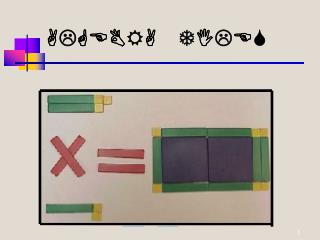DownloadDownload PresentationALGEBRA TILES

# ALGEBRA TILES

Télécharger la présentation## ALGEBRA TILES

- - - - - - - - - - - - - - - - - - - - - - - - - - - E N D - - - - - - - - - - - - - - - - - - - - - - - - - - -
##### Presentation Transcript

1. ALGEBRA TILES

2. ALGEBRA TILES Rachel Magnuson

3. By the end of this Show, you’ll be able to. . . • Define and explain algebra tiles • Be able to use algebra tiles to factor • Show how algebra tiles can model polynomials • Be able to add and subtract polynomials using algebra tiles

4. Why Use Algebra Tiles? • They are inexpensive and widespread • They also make it possible to do the activities that are needed to introduce and explain the distributive law and factoring • Provide a useful way to introduce operations on polynomials • Work extremely well with all ages

5. What are Algebra Tiles? How do they work?

6. Algebra Tiles are manipulatives with which you can represent polynomials and perform polynomial operations, such as adding subtracting, multiplying, and dividing. Each tile represents a specific monomial. Algebra tiles work by the concept that every rectangle has a length, width, and area. The lengths and the widths are the lengths of the sides of the rectangle in some unit. We will be working with unit x. The area is how many squares of that unit it takes to cover the rectangle.

7. Nomials • Monomial – A mathematic expression consisting of a single term. Examples: X, 2Y • Binomial – A mathematic expression consisting of 2 terms connected by a plus or minus sign. Examples: 2X+4, 4Y-2 • Polynomial – A mathematical expression of one or more algebraic terms of which consists of a constant multiplier by one or more variables raise to a non-negative power. Example: 3X2+4x+5 • Trinomial – A polynomial of 3 terms. Example: 2X+4Y-Z

8. Algebra Tiles can be key in understanding factoring. Let’s take a look at factoring.

9. Key Points • You can think of factoring as the reverse of First Outside Inside Last (FOIL) • Factoring is used to break down or factor a quadratic or higher order equation • You can check your factors by using FOIL to determine if you get the original equation back

10. 3 monomials we’ll be working with • Large Square X2

11. The Basics of Algebra Tiles

12. LargeSquares • These are the blocks that will be known as x2.  These blocks appear as blue when positive and red when negative

13. Rectangles • The rectangular blocks that are as long as the square blocks but not as tall are known as x.  These blocks appear as green when positive and red when negative.

14. SmallSquares • The very small squares are constant terms.  They look like the blocks that you probably used in addition and subtraction.  They are numbers like 5, 7 ,9, etc.  These blocks appear as yellow when positive and red when negative.

15. Some Area Examples 5 4 7 9 7 7 (5 x 7) 35 (4 x 9) 36 (7 x 7) 49

16. Now, let’s change the numbers in the previous rectangles to algebraic variables. A Y X B X X (B x A) AB (Y x X) XY (X x X) X2

17. Algebra Tiles Algebra Tiles are a geometric way to factor. Each size and shape tile has a specific value. This value is determined by the size of each side of a rectangle or square. If you recall, the area of a rectangle is length * width, the area of a square is side * side or s2. 1 x 1 1 x 1 1 -1 -1 1 -x -x x2 x -x -x2 x x

18. Examples of tiles used to represent equations such as the following: 1 – x2’s 3 – x’s 2 – 1’s 2 – x2’s 5 – x’s 2 – 1’s

19. In some cases, you can combine these shapes to form perfect rectangles or squares. Here are two examples:

20. Now lets try factoring these equations that are formed into rectangles. The first two examples will be done for you.

21. Resources • Learning about Algebra Tiles • Examples using Algebra Tiles • Everything you need to know • Play with Algebra Tiles online • Make your own Algebra Tiles